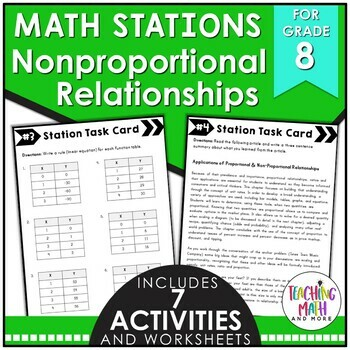## Monday, September 26, 2016

### Teaching Proportional & Non-Proportional Relationships {Grade 8}

There are many different ways to approach teaching Proportional & Non-Proportional Relationships in Math. I love to use the compare and contrast method. This is especially helpful in that it increases learning gains (Marzano Research) and helps students to be able to know the characteristics of both. Here are some helpful resources for developing conceptual knowledge of Proportional & Non-Proportional Relationships.This math station activity is intended to help students understand how to graph proportional relationships, interpret the unit rate as the slope, compare two different proportional relationships, compare properties of two functions, and construct a function to model a linear relationship and determine the rate of change.
Included are:
-6 different stations to engage students
-Teacher facilitated activity for 60-90 minutes of classroom time
-Stations include:
1. Vocabulary
3. Practice of Proportional Relationships
4. Additional Practice of Proportional Relationships
5. Real World applications of Proportional Relationships
6. Word problems of Proportional Relationships
-Student Station Guide helps students record their answersThis math station activity is intended to help students understand how to explain why the slope is the same between any two distinct points, compare properties of two functions, interpret equations as proportional or non-proportional, and construct a function to model a linear relationship.Included are:
-6 different stations to engage students
-Teacher facilitated activity for 60-90 minutes of classroom time
-Stations include:
1. Vocabulary
3. Practice of Non-Proportional Relationships
4. Additional Practice of Non-Proportional Relationships
5. Real World applications of Non-Proportional Relationships
6. Word problems of Non-Proportional Relationships
-Student Station Guide helps students record their answersThese interactive notebook activities are intended to help students understand how to graph proportional relationships, interpret the unit rate as the slope, compare two different proportional relationships, compare properties of two functions, and construct a function to model a linear relationship and determine the rate of change.
Included are:
-4 different Proportional Relationships activities to engage students
-Teacher facilitated activity for 60-90 minutes of classroom time
-Activities include: Vocabulary, Writing a rule (linear equation) from a function with a proportional relationship, Finding the slope between two points, and Writing a function table and drawing a graph from a linear equation.
-Examples of Completed Interactive Math Notebook Activities are included